# Chapter 5 Multivariate Graphs

Multivariate graphs display the relationships among three or more variables. There are two common methods for accommodating multiple variables: grouping and faceting.

## 5.1 Grouping

In grouping, the values of the first two variables are mapped to the x and y axes. Then additional variables are mapped to other visual characteristics such as color, shape, size, line type, and transparency. Grouping allows you to plot the data for multiple groups in a single graph.

Using the Salaries dataset, let’s display the relationship between yrs.since.phd and salary.

library(ggplot2)
data(Salaries, package="carData")

# plot experience vs. salary
ggplot(Salaries,
aes(x = yrs.since.phd,
y = salary)) +
geom_point() +
labs(title = "Academic salary by years since degree")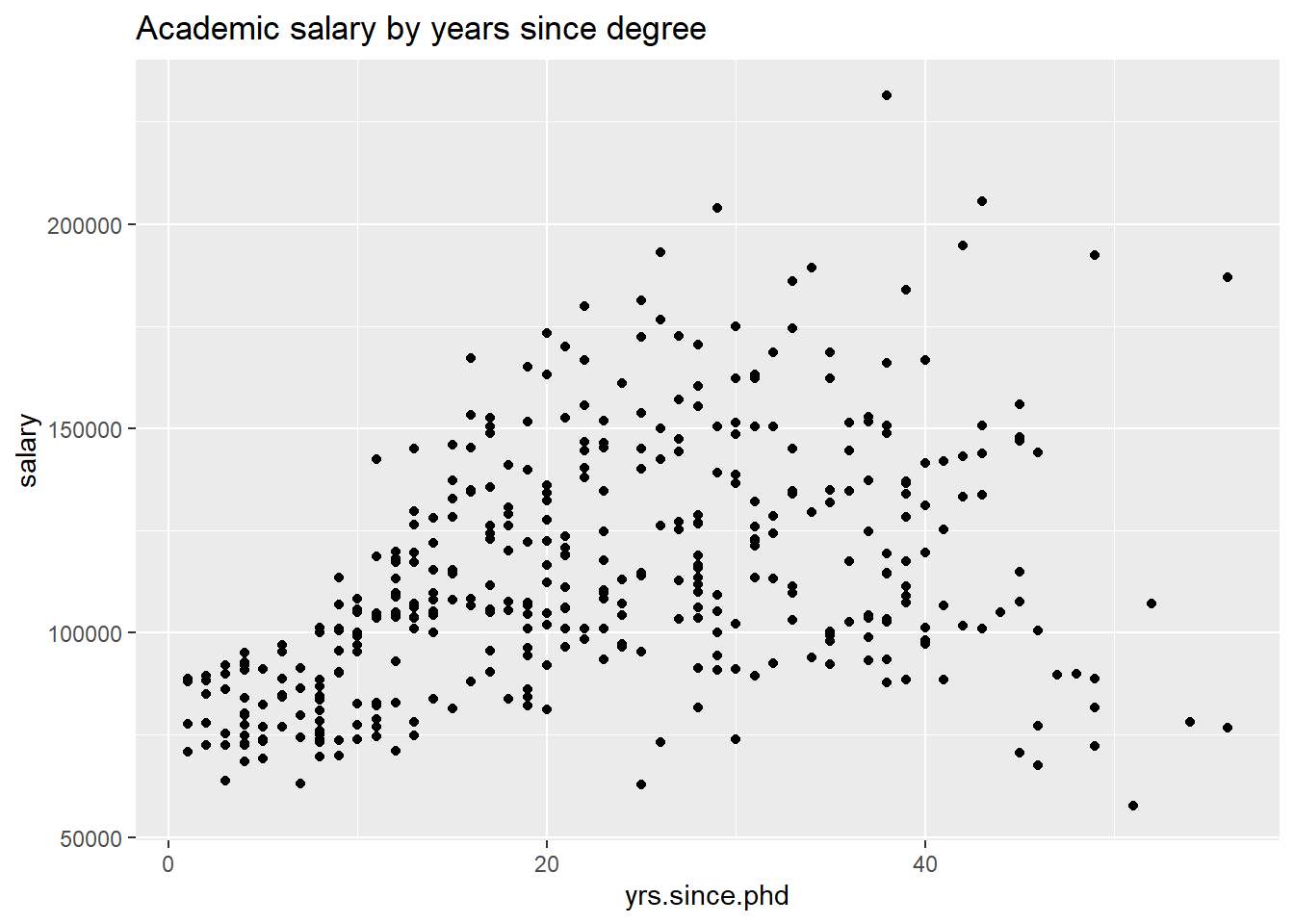Figure 5.1: Simple scatterplot

Next, let’s include the rank of the professor, using color.

# plot experience vs. salary (color represents rank)
ggplot(Salaries, aes(x = yrs.since.phd,
y = salary,
color=rank)) +
geom_point() +
labs(title = "Academic salary by rank and years since degree")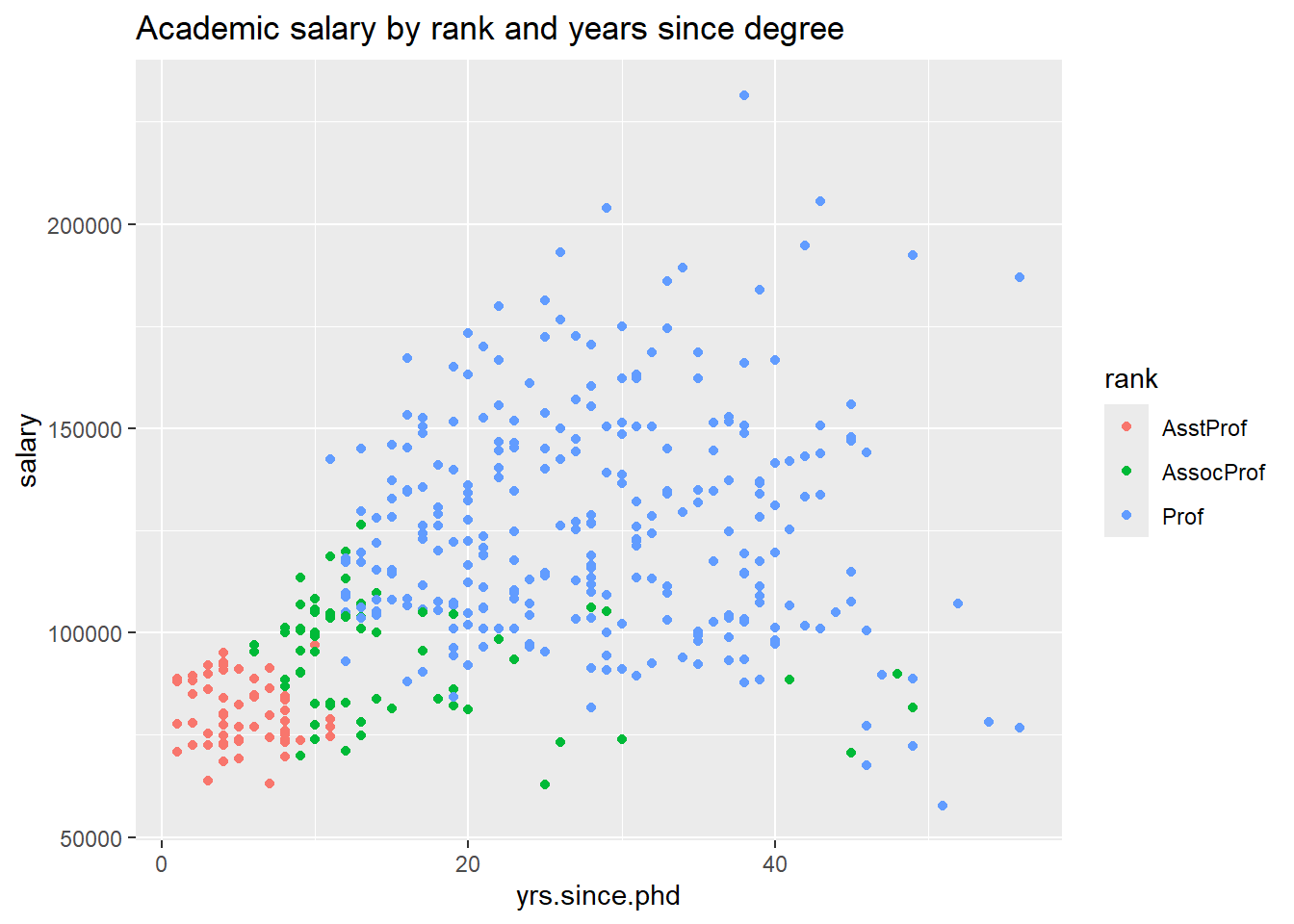Figure 5.2: Scatterplot with color mapping

Finally, let’s add the gender of professor, using the shape of the points to indicate sex. We’ll increase the point size and add transparency to make the individual points clearer.

# plot experience vs. salary
# (color represents rank, shape represents sex)
ggplot(Salaries,
aes(x = yrs.since.phd,
y = salary,
color = rank,
shape = sex)) +
geom_point(size = 3,
alpha = .6) +
labs(title = "Academic salary by rank, sex, and years since degree")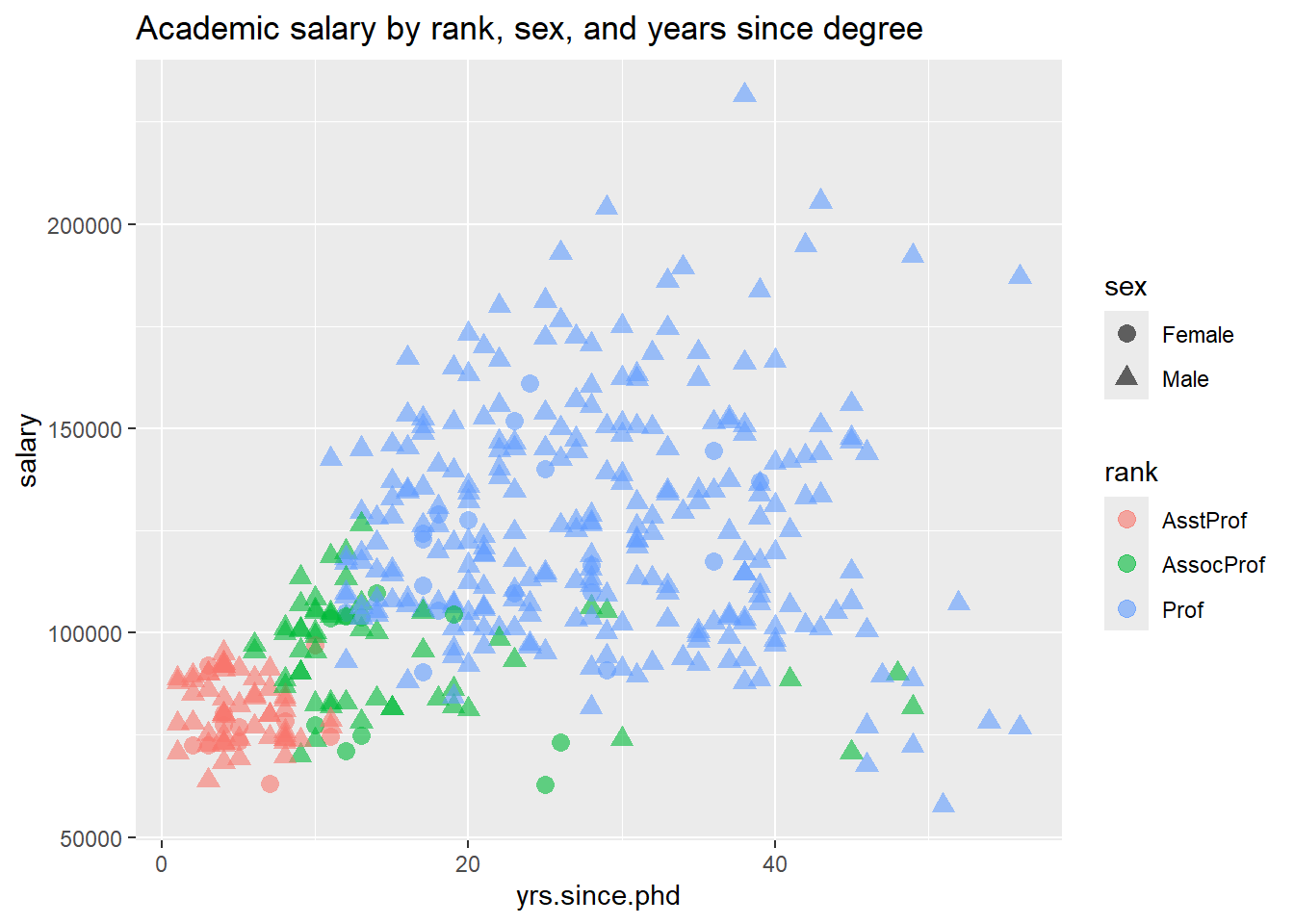Figure 5.3: Scatterplot with color and shape mapping

I can’t say that this is a great graphic. It is very busy, and it can be difficult to distinguish male from female professors. Faceting (described in the next section) would probably be a better approach.

Notice the difference between specifying a constant value (such as size = 3) and a mapping of a variable to a visual characteristic (e.g., color = rank). Mappings are always placed within the aes function, while the assignment of a constant value always appear outside of the aes function.

Here is a cleaner example. We’ll graph the relationship between years since Ph.D. and salary using the size of the points to indicate years of service. This is called a bubble plot.

library(ggplot2)
data(Salaries, package="carData")

# plot experience vs. salary
# (color represents rank and size represents service)
ggplot(Salaries,
aes(x = yrs.since.phd,
y = salary,
color = rank,
size = yrs.service)) +
geom_point(alpha = .6) +
labs(title = "Academic salary by rank, years of service, and years since degree")Figure 5.4: Scatterplot with size and color mapping

There is obviously a strong positive relationship between years since Ph.D. and year of service. Assistant Professors fall in the 0-11 years since Ph.D. and 0-10 years of service range. Clearly highly experienced professionals don’t stay at the Assistant Professor level (they are probably promoted or leave the University). We don’t find the same time demarcation between Associate and Full Professors.

Bubble plots are described in more detail in a later chapter.

As a final example, let’s look at the yrs.since.phd vs salary and add sex using color and quadratic best fit lines.

# plot experience vs. salary with
# fit lines (color represents sex)
ggplot(Salaries,
aes(x = yrs.since.phd,
y = salary,
color = sex)) +
geom_point(alpha = .4,
size = 3) +
geom_smooth(se=FALSE,
method = "lm",
formula = y~poly(x,2),
size = 1.5) +
labs(x = "Years Since Ph.D.",
title = "Academic Salary by Sex and Years Experience",
subtitle = "9-month salary for 2008-2009",
y = "",
color = "Sex") +
scale_y_continuous(label = scales::dollar) +
scale_color_brewer(palette = "Set1") +
theme_minimal()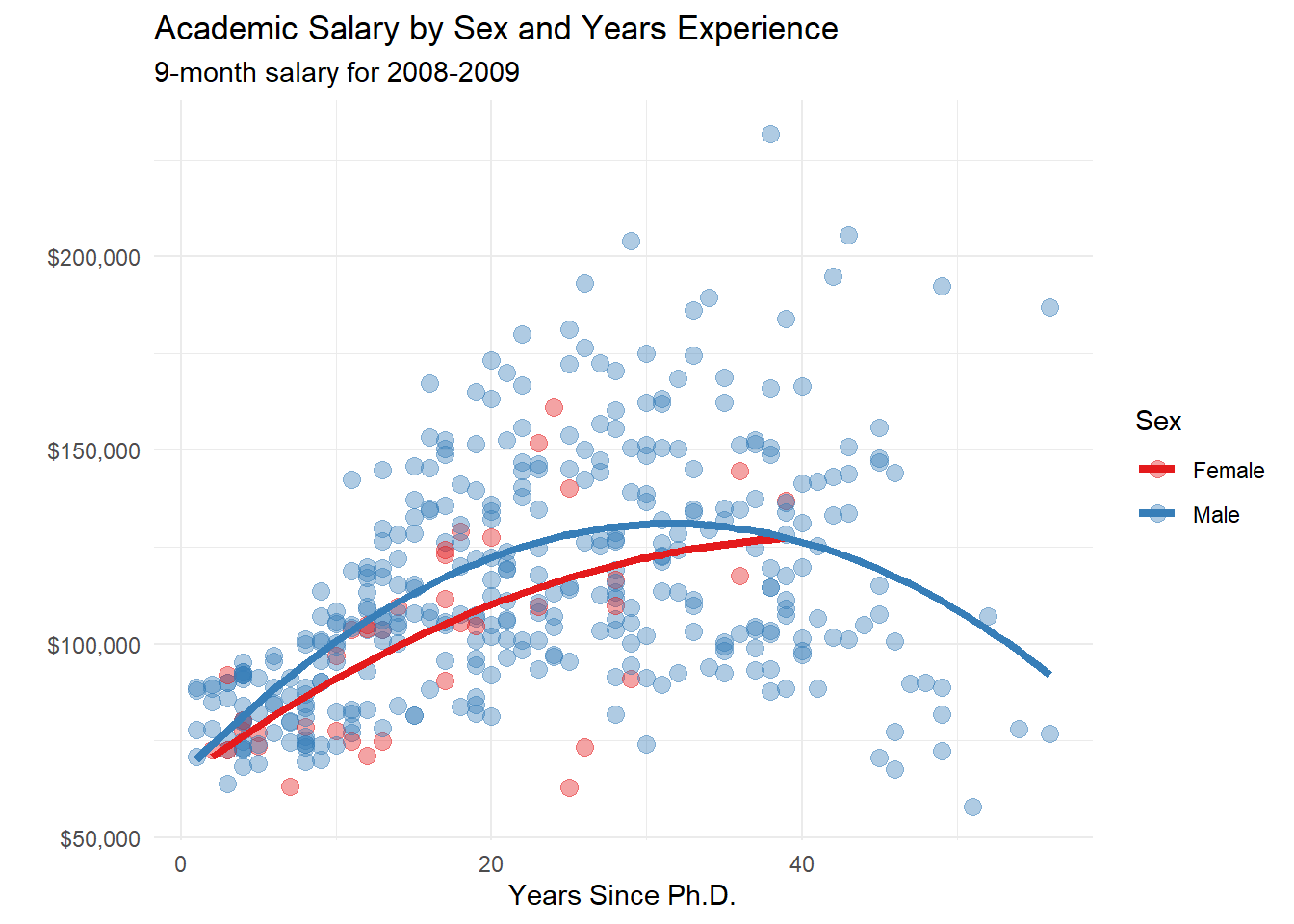Figure 5.5: Scatterplot with color mapping and quadratic fit lines

## 5.2 Faceting

Grouping allows you to plot multiple variables in a single graph, using visual characteristics such as color, shape, and size.

In faceting, a graph consists of several separate plots or small multiples, one for each level of a third variable, or combination of variables. It is easiest to understand this with an example.

# plot salary histograms by rank
ggplot(Salaries, aes(x = salary)) +
geom_histogram(fill = "cornflowerblue",
color = "white") +
facet_wrap(~rank, ncol = 1) +
labs(title = "Salary histograms by rank")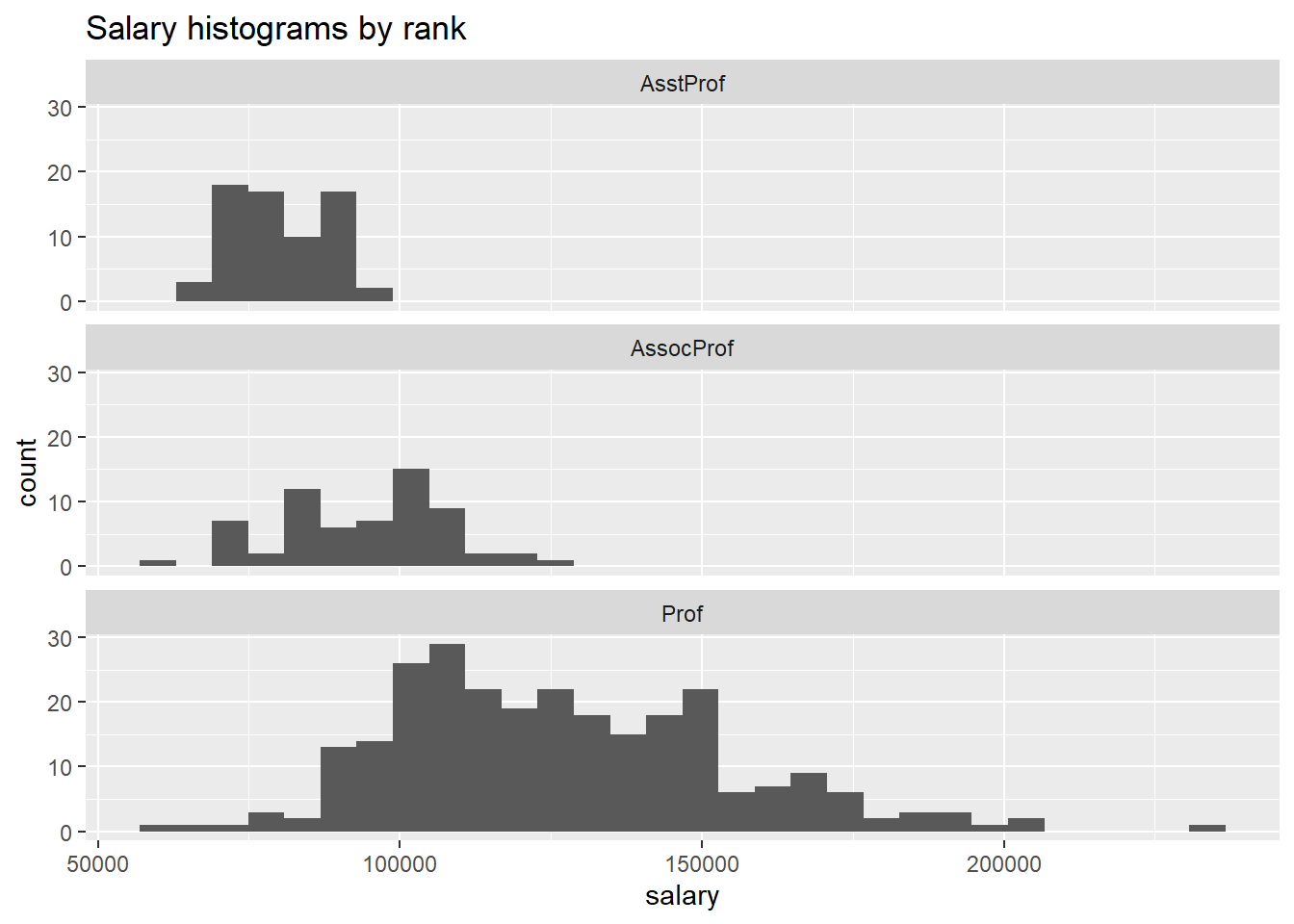Figure 5.6: Salary distribution by rank

The facet_wrap function creates a separate graph for each level of rank. The ncol option controls the number of columns.

In the next example, two variables are used to define the facets.

# plot salary histograms by rank and sex
ggplot(Salaries, aes(x = salary / 1000)) +
geom_histogram(color = "white",
fill = "cornflowerblue") +
facet_grid(sex ~ rank) +
labs(title = "Salary histograms by sex and rank",
x = "Salary ($1000)")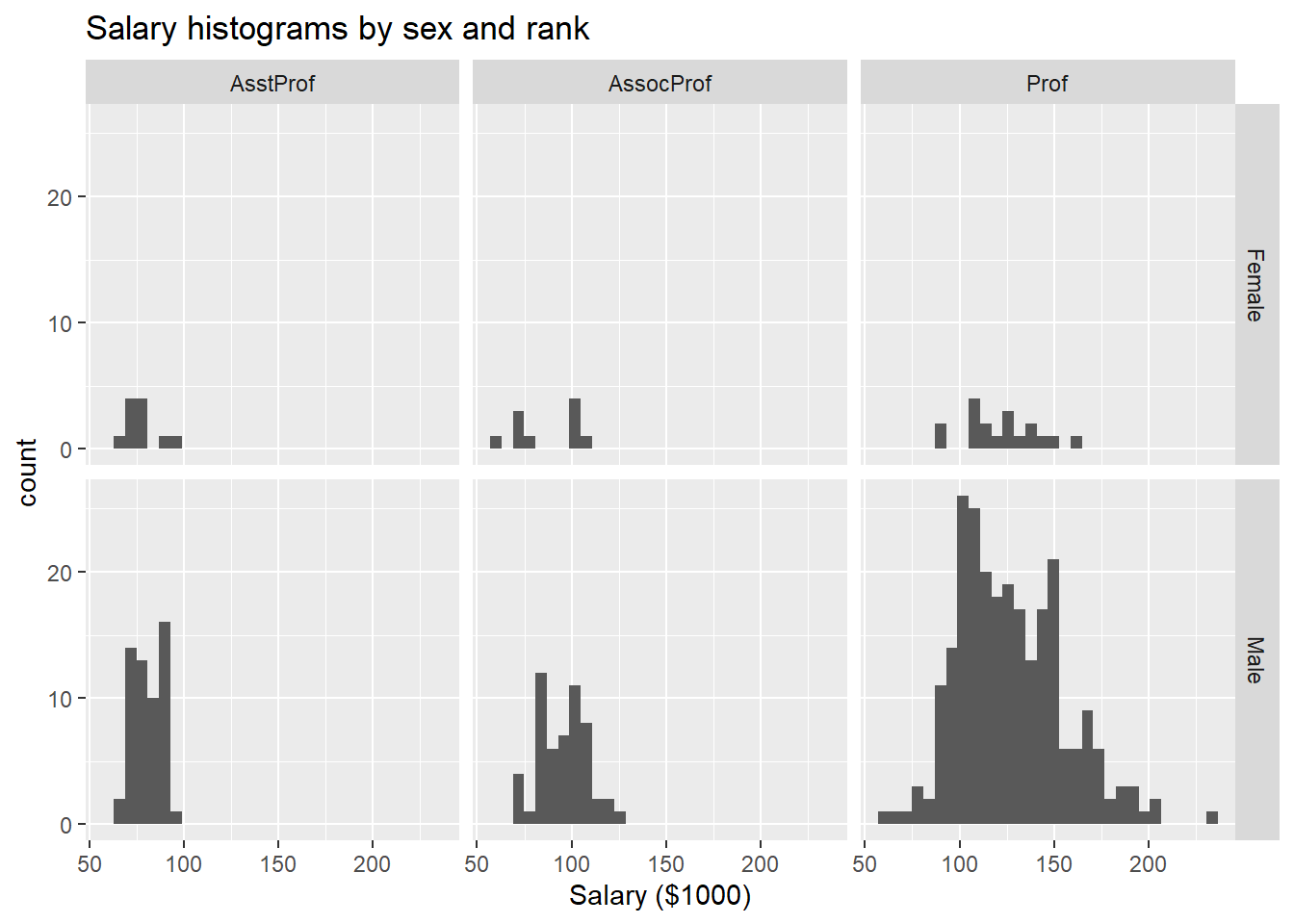Figure 5.7: Salary distribution by rank and sex The format of the facet_grid function is facet_grid( row variable(s) ~ column variable(s)) Here, the function assigns sex to the rows and rank to the columns, creating a matrix of 6 plots in one graph. We can also combine grouping and faceting. Let’s use Mean/SE plots and faceting to compare the salaries of male and female professors, within rank and discipline. We’ll use color to distinguish sex and faceting to create plots for rank by discipline combinations. # calculate means and standard erroes by sex, # rank and discipline library(dplyr) plotdata <- Salaries %>% group_by(sex, rank, discipline) %>% summarize(n = n(), mean = mean(salary), sd = sd(salary), se = sd / sqrt(n)) # create better labels for discipline plotdata$discipline <- factor(plotdata\$discipline,
labels = c("Theoretical",
"Applied"))
# create plot
ggplot(plotdata,
aes(x = sex,
y = mean,
color = sex)) +
geom_point(size = 3) +
geom_errorbar(aes(ymin = mean - se,
ymax = mean + se),
width = .1) +
scale_y_continuous(breaks = seq(70000, 140000, 10000),
label = scales::dollar) +
facet_grid(. ~ rank + discipline) +
theme_bw() +
theme(legend.position = "none",
panel.grid.major.x = element_blank(),
panel.grid.minor.y = element_blank()) +
labs(x="",
y="",
title="Nine month academic salaries by gender, discipline, and rank",
subtitle = "(Means and standard errors)") +
scale_color_brewer(palette="Set1")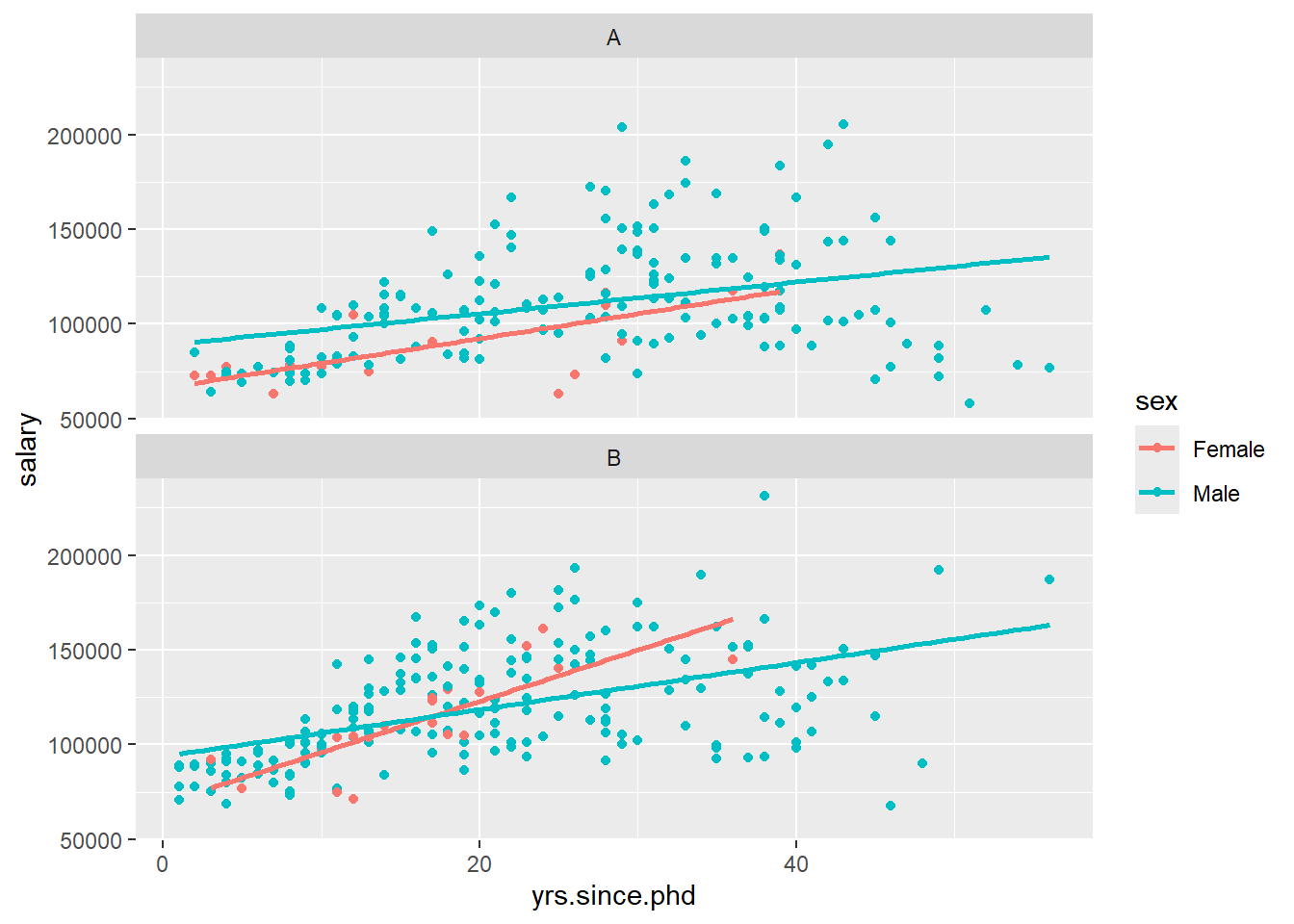Figure 5.8: Salary by sex, rank, and discipline

The statement facet_grid(. ~ rank + discipline) specifies no row variable (.) and columns defined by the combination of rank and discipline.

The theme_ functions create create a black and white theme and eliminates vertical grid lines and minor horizontal grid lines. The scale_color_brewer function changes the color scheme for the points and error bars.

At first glance, it appears that there might be gender differences in salaries for associate and full professors in theoretical fields. I say “might” because we haven’t done any formal hypothesis testing yet (ANCOVA in this case).

As a final example, we’ll shift to a new dataset and plot the change in life expectancy over time for countries in the “Americas”. The data comes from the gapminder dataset in the gapminder package. Each country appears in its own facet. The theme functions are used to simplify the background color, rotate the x-axis text, and make the font size smaller.

# plot life expectancy by year separately
# for each country in the Americas
data(gapminder, package = "gapminder")

# Select the Americas data
plotdata <- dplyr::filter(gapminder,
continent == "Americas")

# plot life expectancy by year, for each country
ggplot(plotdata, aes(x=year, y = lifeExp)) +
geom_line(color="grey") +
geom_point(color="blue") +
facet_wrap(~country) +
theme_minimal(base_size = 9) +
theme(axis.text.x = element_text(angle = 45,
hjust = 1)) +
labs(title = "Changes in Life Expectancy",
x = "Year",
y = "Life Expectancy")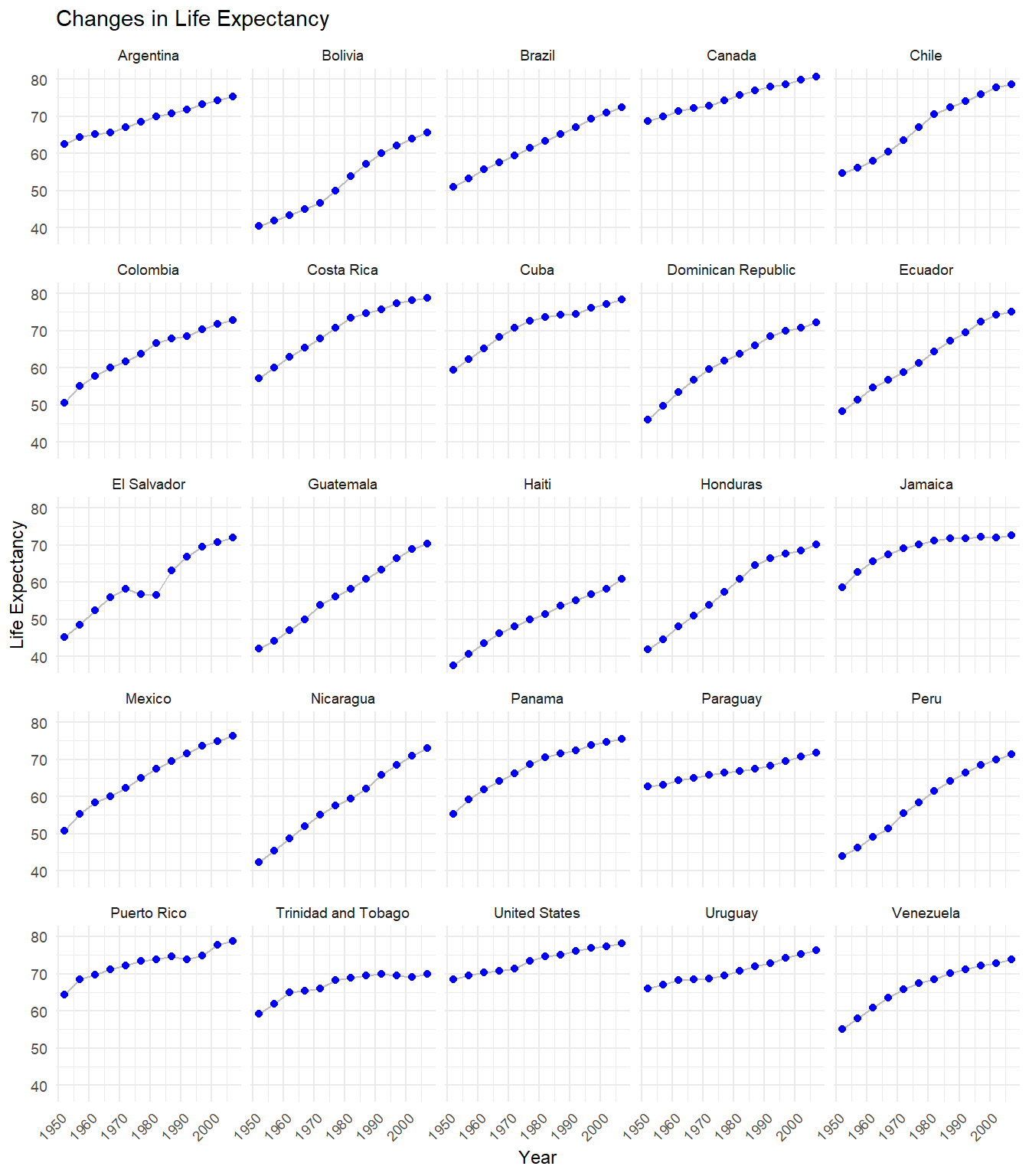Figure 5.9: Changes in life expectancy by country

We can see that life expectancy is increasing in each country, but that Haiti is lagging behind.